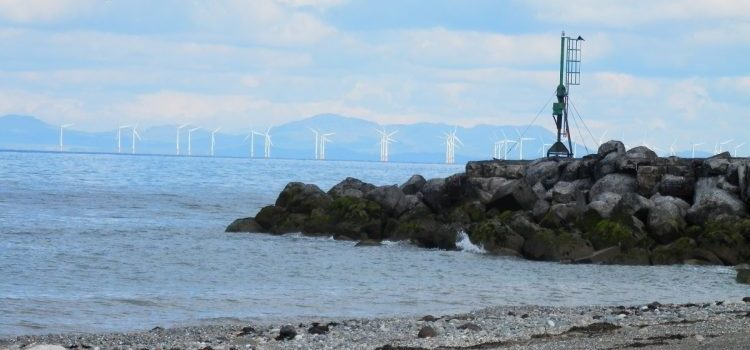# Fundamentals of Wind PowerWind turbines have been used to generate electrical power for over 150 years [see the history of windpower], but how do they work?

In order to explain how wind turbines operate it is easier if they are first broken down into two different systems:

• 1) the mechanical system (the main moving parts), this determines the overall output of the wind turbine
• 2) the electrical system which is responsible for converting all of the energy harvested by the mechanical system into electricity that you can use in your house.
##### The mechanical system

When you look at a wind turbine you can see all of the main components that make up the mechanical system as labeled on the picture below

The main job of these parts of a wind turbine is to take the energy that is inside a moving volume of air and turn it into movement of the turbine. Therefore it is important to consider the energy involved.

The energy contained within the moving air can be worked out by taking the total mass of air that passes through the turbine in a set amount of time and then working out its kinetic energy using the following equation:

Energy in the air = 1/2 the mass of the air × the speed of the air × the speed of the air

This is great but most people don’t know the mass of a passing gust of wind, but this isn’t a problem because if we know how fast the air is moving and how big the wind turbine is it can worked out.

The first the area that the wind turbine is collecting the air from is determined. In order to do this the length of one of the rotors (or the radius of the circle is used in this equation).

Area of turbine = length of rotor blade × length of rotor blade ×π

The constant value  in the above equation is used when working out areas and volumes related to circles and has a value of just over three.

Taking this area it is then possible to determine the volume that passes through the turbine in a second, using the following equation:

Volume per second= Area of turbine × speed of air

This equation will provide the total volume of air that passes through the turbine.

Come back later to find out more!